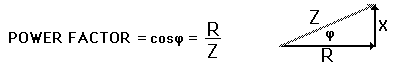Home » Posts tagged 'Power'

# Tag Archives: Power

## Wave particle duality

Let n and m denote the number of photons emitted by a red and blue bulb respectively of equal power in a given time.Is n=m?

Answer:

No

The energy of a photon is hν

where ν is the frequency.

Power = energy/time

==> nhν1 = mhν2

==> nν1 = mν2

clearly n not equal to m

## Power Factor

Divya asked:

“A COIL  HAS  A POWER  FACTOR OF 0.707 AT 60Hz, THEN ITS  POWER  FACTOR AT 180Hz WILL BE- ……….?

Ans:

(to be posted soon)

## A Problem from power

Gayathri Shinde Asks:

“Please answer this question with explanation:

The power output of a machine that lifts a 600 kg
weight through a height of 20m in 1min is:
@)1.96kW    b)0.98kW

c)12kW      d)3.92kW”

Answer:

Use the formula power = work /time$P = \frac{mgh}{t}$
[youtube=http://www.youtube.com/watch?v=BVxEEn3w688&rel=0&w=480&h=390]

## Power factor in AC circuits

Dany Asked:

“what is meant by power factor?”

Ans:

In AC circuits, Power factor is defined as the ratio of true power to apparent power.$Power Factor =\frac{True Power}{Apparent Power}$

If φ is the phase difference between E and I, then

True Power = EvIv cos φ and the Apparent Power is EvIv

Therefore,### Visitors So Far @ AskPhysics

• 2,115,584 hits

### Subscribe to Blog via Email

Enter your email address to subscribe to this blog and receive notifications of new posts by email.

Join 4,004 other subscribers

### askphysics@TwitterAsk Physics
@askphysics